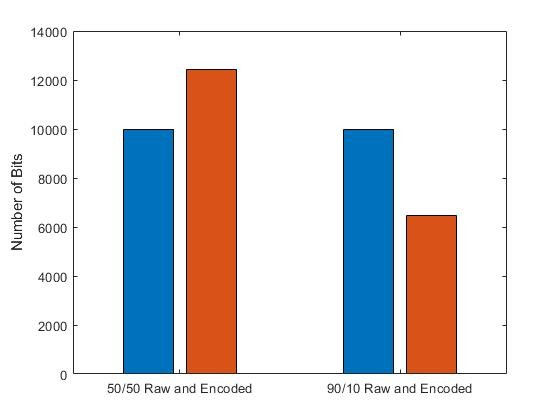This part is from Chapter 4.1.1 (SDR 4 Engineers) (pg 121-122)

One of the goals of any communication system is to efficiently and reliably communicate information across a medium from a transmitter to a receiver. Source encoding is a mechanism designed to remove redundant information in order to facilitate more efficient communications.

The way source encoding operates is by taking a sequence of source symbolsand mapping them to a corresponding sequence of source encoded symbolsas close to random as possible and the components ofare uncorrelated (i.e., unrelated).

In other words, a source encoder removes redundant information from the source symbols in order to realize efficient transmission. Note that in order to perform source encoding, the source symbols need to be digital.

In the following MATLAB script, we generate two binary data vectors, with one possessing an equal amount of one and zero values while the other vector possesses approximately 90% one values and 10% zero values. To compress the data in these vectors, we use an encoding technique where we take all continuous strings of ones in each vector and replace it with a decimal value representing the length of these strings of one. For example, if a binary vector existed, and 15 one values exist between two zero values, we would replace those 15 one values with a decimal value (or equivalent codeword) indicating that 15 one values exist in that part of the binary vector.

Based on the implemented encoding scheme, our intuition would dictate that the binary vector with the 90/10 ratio of one to zero values would be compressed more relative to the binary vector with the 50/50 ratio since the former would have a greater likelihood of having long strings of one values to compress. Referring to the figure below, our intuition is confirmed, with a significant reduction in size for the compressed 90/10 binary data stream. On the other hand, we observe that the 50/50 binary vector does not even compress but rather grow in size. This is due to the fact that the amount of overhead needed to replace every string of one values with a corresponding codeword actually takes up more information than the original binary sequence. Consequently, when performing source coding, it is usually worth our while to compress data streams that possess obvious amounts of redundancy, otherwise we can actually make the situation even more inefficient.

Output: